# An Introduction to ClassifyR

Dario Strbenac, John Ormerod, Jean Yang
The University of Sydney, Australia.

## Overview

ClassifyR provides two contributions. Firstly, there is a structured pipeline for two-class classification. Classification is viewed in terms of four stages, data transformation, feature selection, classifier training, and prediction. The stages can be run in any order that is sensible. Each step can be provided with functions that follow some rules about parameters. Additionally, the driver function implements resampling with replacement k-fold cross validation as well as leave k out cross validation. This function can use parallel processing capabilities in R to speed up cross validations when many CPUs are available. Some convenience function interfaces are provided for microarray and RNA-seq data, while other functions work directly with the framework without the need for an interface.

Secondly, it implements a number of methods for classification using different feature types. Most classifiers work with features where the means are different. In addition to differential expression, ClassifyR also considers differential deviation and differential distribution.

The function that drives the classification is runTests. For cross validation, it repeatedly calls runTest, which runs a classification for a single split of the data.

In the following sections, the functions provided in ClassifyR will be demonstrated. However, a user can provide any function to the classification framework, as long as it meets some minimal rules. See the last section “Rules for New Functions” for a description of these.

## Case Study : Survival for Ovarian Cancer Patients.

A survival study was performed on microarrays for ovarian cancers and is available from curatedOvarianData on Bioconductor. Load the dataset into the current R session. Only 1000 genes are used for illustration.

``````library(ClassifyR)
``````
``````## Loading required package: Biobase
##
## Attaching package: 'BiocGenerics'
##
## The following objects are masked from 'package:parallel':
##
##     clusterApply, clusterApplyLB, clusterCall, clusterEvalQ, clusterExport, clusterMap, parApply, parCapply,
##     parLapply, parLapplyLB, parRapply, parSapply, parSapplyLB
##
## The following object is masked from 'package:stats':
##
##     xtabs
##
## The following objects are masked from 'package:base':
##
##     Filter, Find, Map, Position, Reduce, anyDuplicated, append, as.data.frame, as.vector, cbind, colnames, do.call,
##     duplicated, eval, evalq, get, intersect, is.unsorted, lapply, mapply, match, mget, order, paste, pmax, pmax.int,
##     pmin, pmin.int, rank, rbind, rep.int, rownames, sapply, setdiff, sort, table, tapply, union, unique, unlist,
##     unsplit
##
## Welcome to Bioconductor
##
##     Vignettes contain introductory material; view with 'browseVignettes()'. To cite Bioconductor, see
##     'citation("Biobase")', and for packages 'citation("pkgname")'.
##
``````
``````library(curatedOvarianData)
``````
``````## Loading required package: affy
``````
``````data(GSE26712_eset)
GSE26712_eset <- GSE26712_eset[1:1000, ]
``````

Define patients who died less than 1 years as poor outcomes, and those that survived more than 5 years as good outcomes.

``````curatedClinical <- pData(GSE26712_eset)
ovarPoor <- curatedClinical[, "vital_status"] == "deceased" & curatedClinical[, "days_to_death"] < 365 * 1
ovarGood <- curatedClinical[, "vital_status"] == "living" & curatedClinical[, "days_to_death"] > 365 * 5
sum(ovarPoor, na.rm = TRUE)
sum(ovarGood, na.rm = TRUE)
``````
``````##  27
##  20
``````

There are 27 poor prognosis patients and 20 good prognosis patients. The expression data is subset to only keep patients in the Poor or Good group.

``````ovarExpression <- exprs(GSE26712_eset)[, c(which(ovarPoor), which(ovarGood))]
ovarGroups <- factor(rep(c("Poor", "Good"), c(length(which(ovarPoor)), length(which(ovarGood)))),
levels = c("Poor", "Good"))
``````

Boxplots are drawn to get an idea of the distrbution of the data.

``````library(ggplot2)
plotData <- data.frame(expression = as.numeric(ovarExpression),
sample = factor(rep(1:ncol(ovarExpression), each = nrow(ovarExpression))))

ggplot(plotData, aes(x = sample, y = expression)) + geom_boxplot() +
scale_y_continuous(limits = c(0, 15)) + xlab("Sample") + ylab("Expression Value") +
ggtitle("Expression for All Arrays")
``````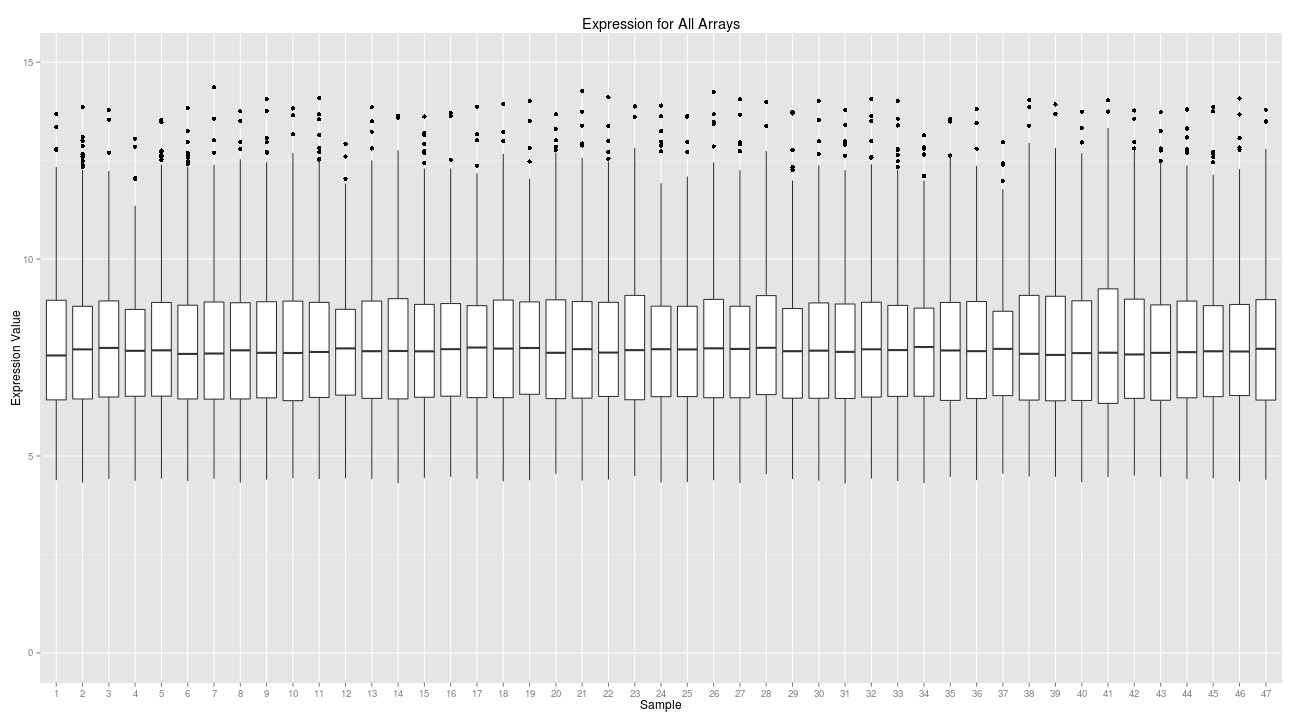All functions provided in ClassifyR work with either a matrix and class vector or an ExpressionSet object. Here, an ExpressionSet object is used.

``````groupsTable <- data.frame(class = ovarGroups)
rownames(groupsTable) <- colnames(ovarExpression)
ovarSet <- ExpressionSet(ovarExpression, AnnotatedDataFrame(groupsTable))
featureNames(ovarSet) <- rownames(ovarExpression)
dim(ovarSet)
``````
``````## Features  Samples
##     1000       47
``````

### Differential Expression

Differential expression classifiers look for consistent changes in means between groups. This is the most common form of classification. Here, a feature selection based on a ranked list from limma followed by a DLDA classifier will be used to do 10 resamples and four folds of cross validation.

``````library(sparsediscrim)
DEresults <- runTests(ovarSet, "Ovarian Cancer", "Differential Expression", validation = "bootstrap", resamples = 5, folds = 3,
params = list(SelectionParams(limmaSelection, resubstituteParams = ResubstituteParams(nFeatures = c(25, 50, 75, seq(100, 1000, 100)), performanceType = "balanced", better = "lower")),
TrainParams(dlda, TRUE, doesTests = FALSE),
PredictParams(predict, TRUE, FALSE, getClasses = function(result) result[["class"]])),
parallelParams = bpparam(), verbose = 1)
DEresults
``````
``````## An object of class 'ClassifyResult'.
## Dataset Name: Ovarian Cancer.
## Classification Name: Differential Expression.
## Validation: 3 fold cross-validation of 5 resamples.
## Predictions: List of data frames of length 5.
## Features: List of length 5 of lists of length 3 of row indices.
## Performance Measures: None calculated yet.
``````

For computers with more than 1 CPU, the number of cores to use can be given to runTests by using the argument parallelParams.

This example introduces the classes SelectionParams, TrainParams, and PredictParams. They store details about the functions and the parameters they use for selection, training, and prediction. The first argument to their constructors is always a function, followed by other arguments. Any named arguments can be provided, if the function specified to the constructor knows how to use an argument of that name. The order in which they are specified in the list determines the order the stages are run in.

The limmaSelection function specified to selectionParams ranks probes based on p-value and uses the classifier specified for trainParams and calculates the resubstitution error rate for the top nFeatures, picking the value with the lowest error rate.

TrainParams has three mandatory arguments. The first is the function that trains a classifier. The second is a logical value that specifies whether expression should be transposed, before being passed to the classifier function. Many classification functions in existing R packages in the CRAN repository need the features to be the columns and samples to be the rows. In ClassifyR, the expression data that is passed to runTests or runTest must have features as rows and samples as columns. This is more common in bioinformatics. In this example, the function dlda expects columns to be features, so transposeExpression is TRUE. Another common difference between classifiers on CRAN is that some of them do training and testing separately, whereas in other packages, one function does training and testing. In the case of dlda, it only does training, so doesTests, the third argument to the constructor, is set to FALSE.

PredictParams has two mandatory arguments. The first is a function which takes a built classifier and does predictions on unseen data. The second is a function which extracts a vector of predicted class labels, from the object returned from the function. In this case, the predict method returns an object which stores predictions in a list element called class.

The top five probes selected in the feature selection step can be checked visually. DEresults is a ClassifyResult object returned by runTests. features is a function that allows access to the row indices that were chosen for each fold.

``````plotFeatureClasses(ovarSet, features(DEresults)[][][1:5])
``````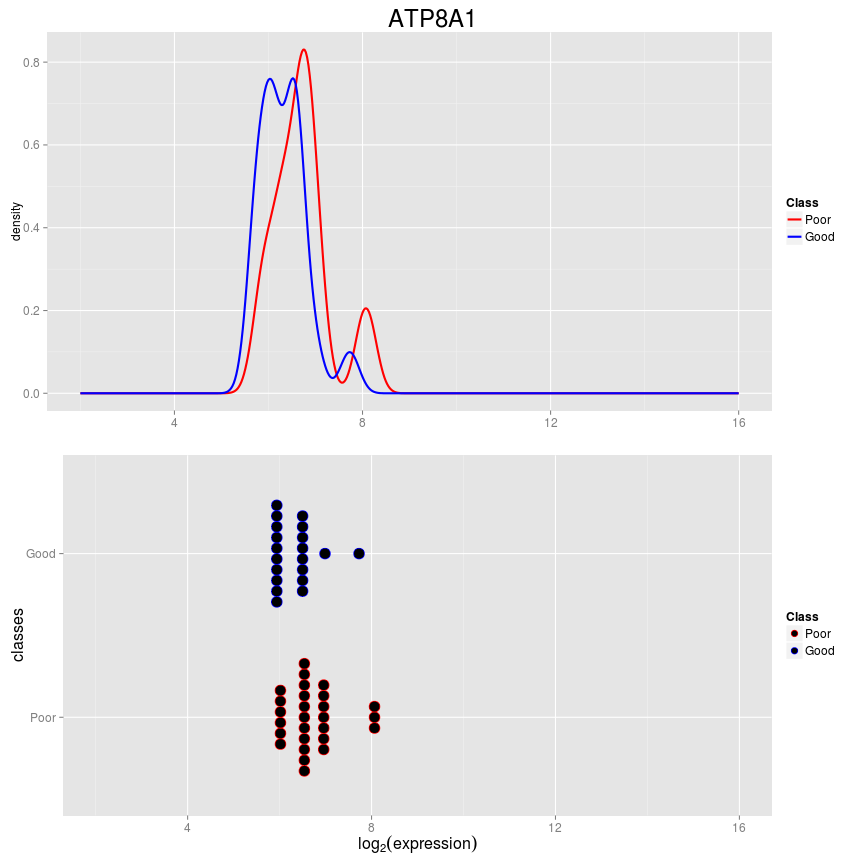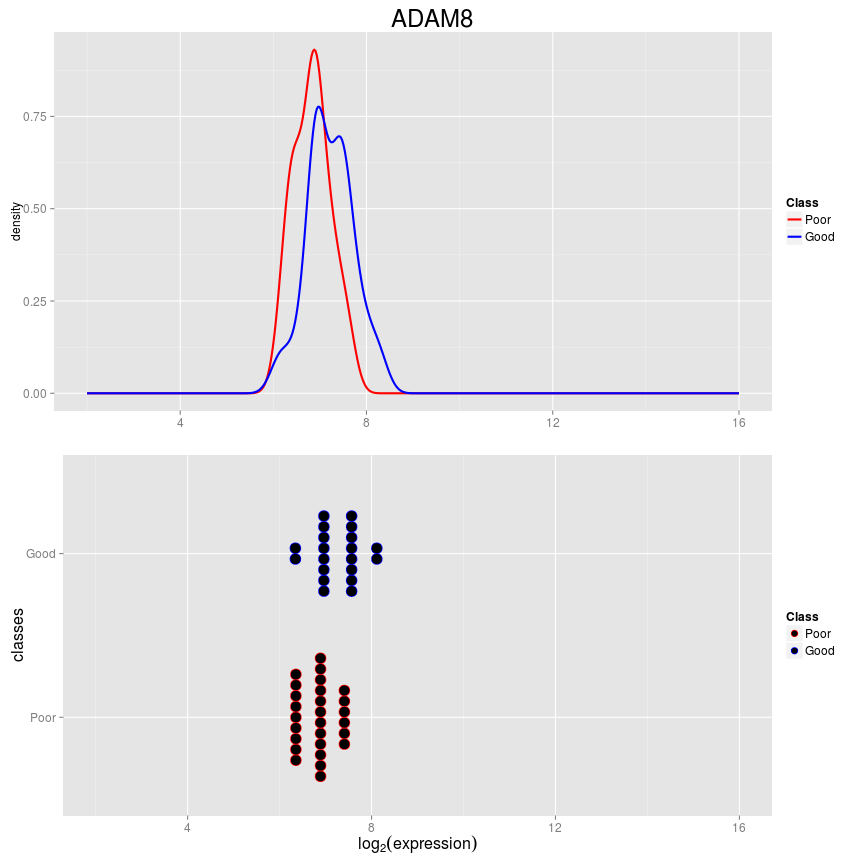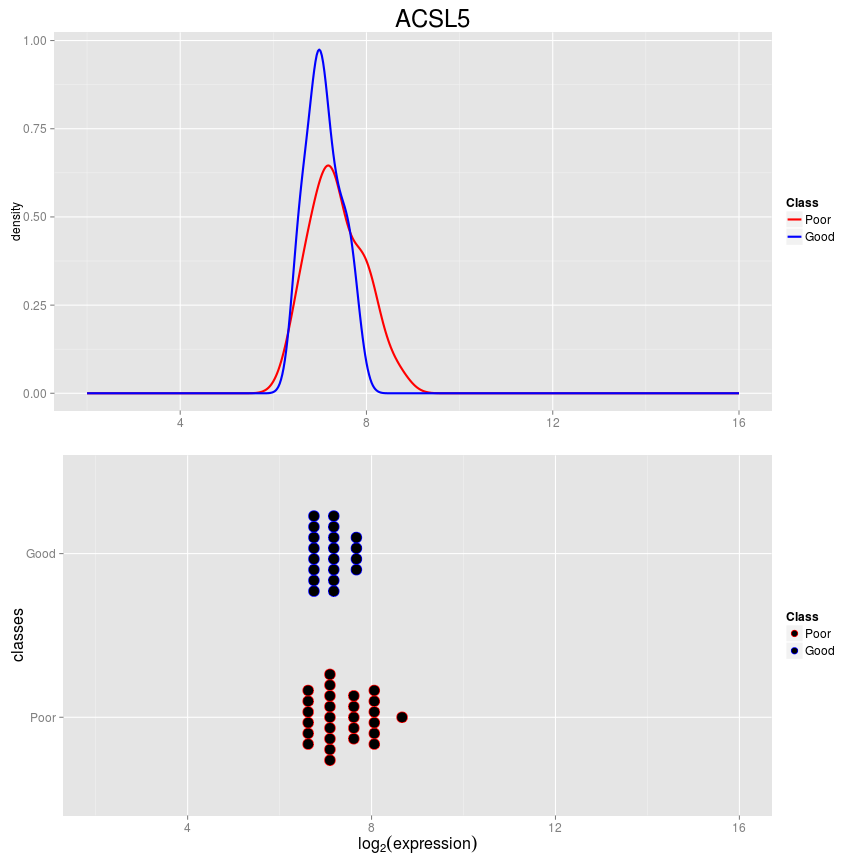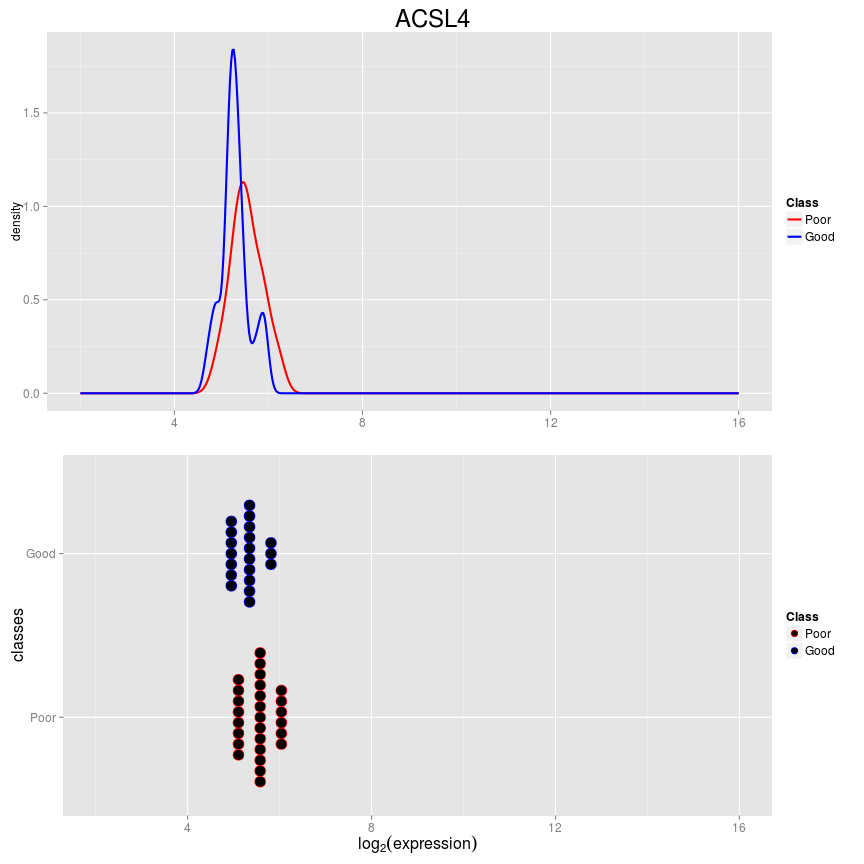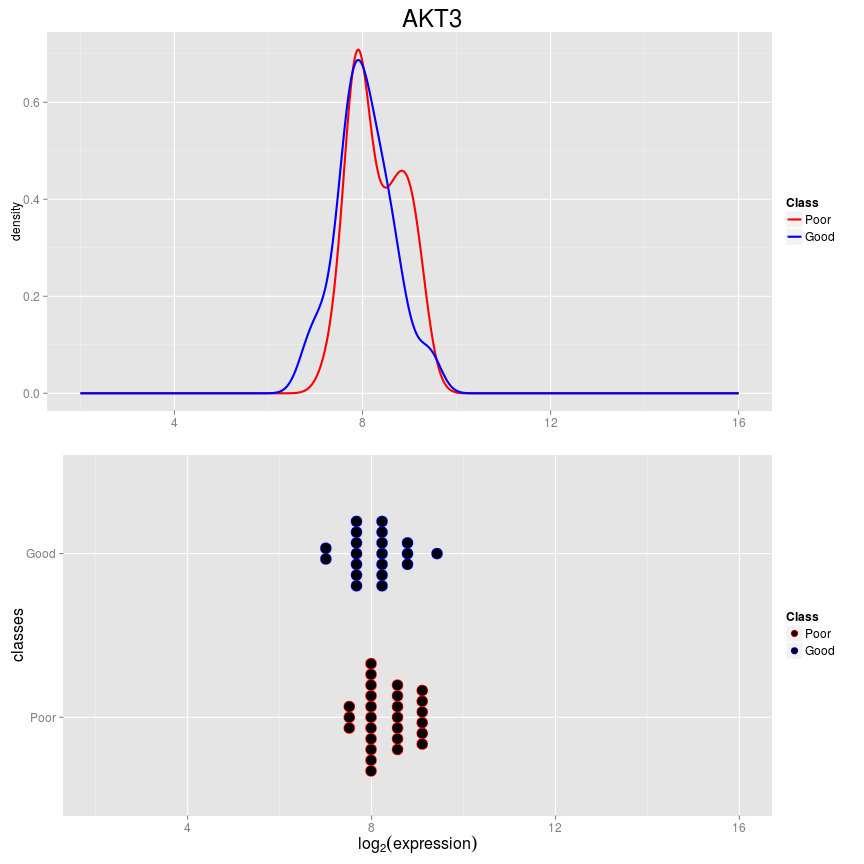This plots the distribution of microarray intensities for the two survival classes for features that were chosen in the first fold of the first resampling. As seen, the means of the probes are not much different.

Classification error rates, as well as many other prediction performance measures, can be calculated with calcPerformance. Next, the balanced error rate is calculated for all ten resamplings. The balanced error rate is defined as the average of the classification errors of each class.

``````DEresults <- calcPerformance(DEresults, "balanced")
DEresults
``````
``````## An object of class 'ClassifyResult'.
## Dataset Name: Ovarian Cancer.
## Classification Name: Differential Expression.
## Validation: 3 fold cross-validation of 5 resamples.
## Predictions: List of data frames of length 5.
## Features: List of length 5 of lists of length 3 of row indices.
## Performance Measures: Balanced Error Rate.
``````
``````performance(DEresults)
``````
``````## \$`Balanced Error Rate`
##  0.2554348 0.3072727 0.3739583 0.4523810 0.3166667
``````

The error rates are reasonable. Any performance measure can be calculated that the ROCR function performance calculates. For example, the Matthews correlation coefficient can also be calculated.

``````DEresults <- calcPerformance(DEresults, "mat")
DEresults
``````
``````## An object of class 'ClassifyResult'.
## Dataset Name: Ovarian Cancer.
## Classification Name: Differential Expression.
## Validation: 3 fold cross-validation of 5 resamples.
## Predictions: List of data frames of length 5.
## Features: List of length 5 of lists of length 3 of row indices.
## Performance Measures: Balanced Error Rate, Matthews correlation coefficient.
``````
``````performance(DEresults)
``````
``````## \$`Balanced Error Rate`
##  0.2554348 0.3072727 0.3739583 0.4523810 0.3166667
##
## \$`Matthews correlation coefficient`
##  0.48913043 0.40589498 0.24455676 0.09064557 0.36265926
``````

ClassifyR has two feature selection functions for differential expression.

• limmaSelection
• edgeRSelection

limmaSelection is suited to microarray data and edgeRSelection is suited to RNA-seq data where the expression values are raw counts.

### Differential Variability

Some diseases are typified not by a change in expression means of features between groups, but a change in the expression variability. This can be observed by when the variance of a gene's expression changes drastically between conditions, such as healthy cells and cancerous cells.

Only two resamples are done.

``````DVresults <- runTests(ovarSet, "Ovarian Cancer", "Differential Deviation",
validation = "bootstrap", resamples = 2, folds = 4,
params = list(SelectionParams(leveneSelection, resubstituteParams = ResubstituteParams(nFeatures = c(25, 50, 75, seq(100, 1000, 100)), performanceType = "balanced", better = "lower")),
TransformParams(subtractFromLocation, location = "median"),
TrainParams(fisherDiscriminant, FALSE, doesTests = TRUE),
PredictParams(predictor = function(){}, FALSE, FALSE, getClasses = function(result) result)),
verbose = 1)
DVresults
``````
``````## An object of class 'ClassifyResult'.
## Dataset Name: Ovarian Cancer.
## Classification Name: Differential Deviation.
## Validation: 4 fold cross-validation of 2 resamples.
## Predictions: List of data frames of length 2.
## Features: List of length 2 of lists of length 4 of row indices.
## Performance Measures: None calculated yet.
``````

For params, the SelectionParams object is specified first. In this analysis, feature selection is done first. A TransformParams object is next in the list, so data transformation will be applied after feature selection has been done. transformParams specifies subtractFromLocation as the transformation funcion. This is because it is anticipated that subtracting all features from the median of the training set will be a good feature to detect differential deviation. trainParams specifies fisherDiscriminant as the classifier function. Note that this function does both training and prediction, so the third parameter is TRUE. predictParams specifies an empty function as the prediction function, because fisherDiscriminant does both steps. fisherDiscriminant directly returns a vector of predictions, so the second function simply returns the argument result.

The top five probes selected for the first resampling and first fold are visualised.

``````plotFeatureClasses(ovarSet, features(DVresults)[][][1:5])
``````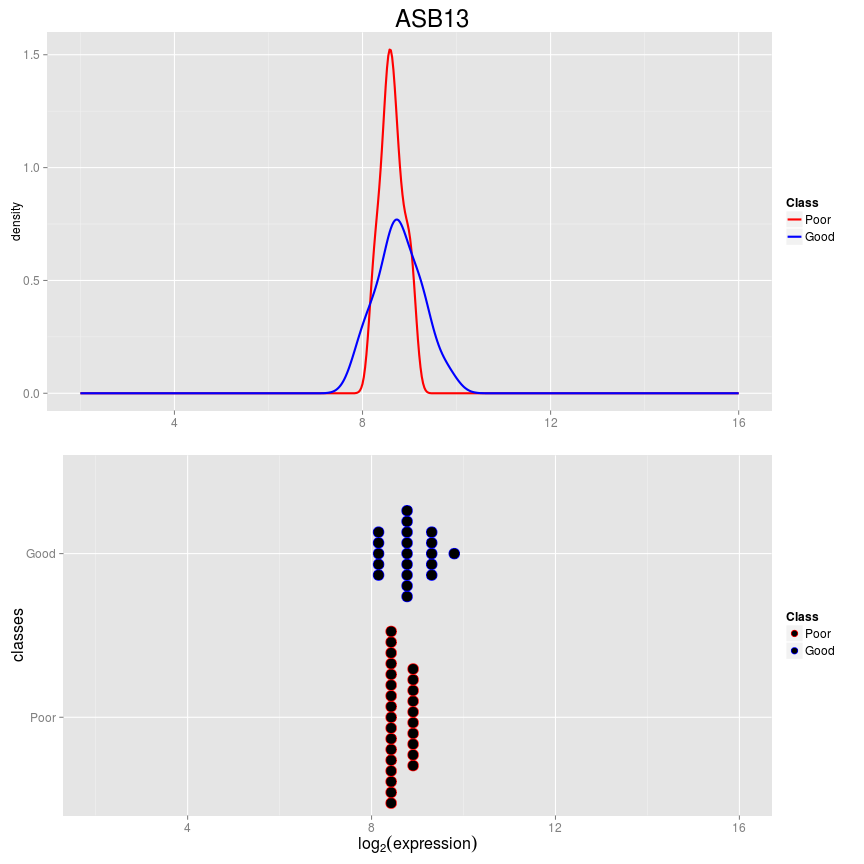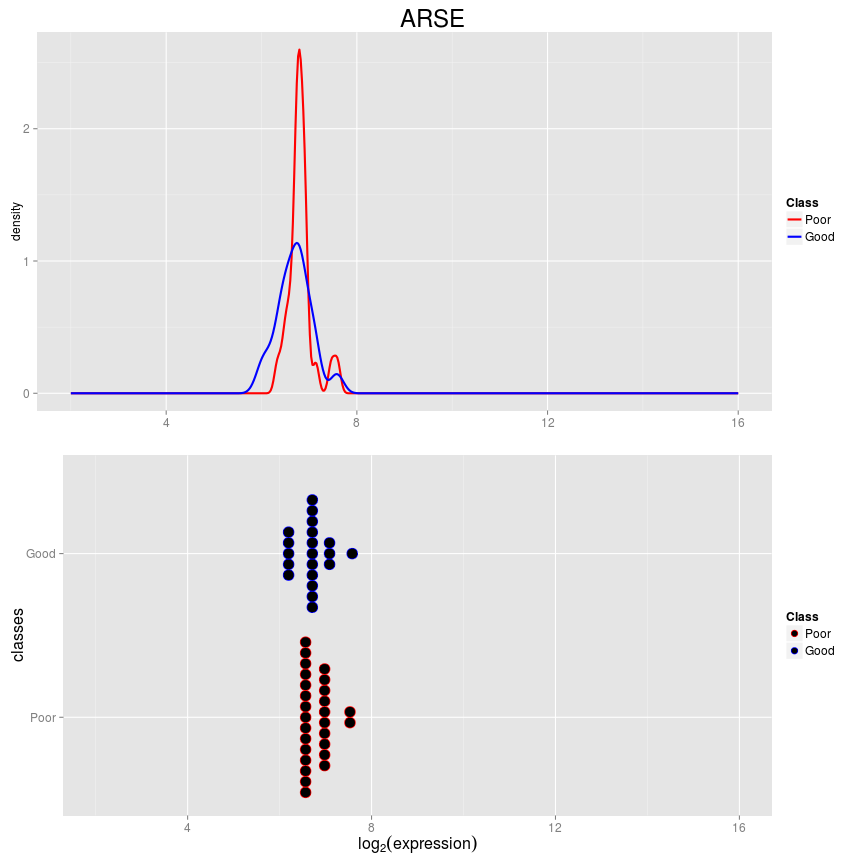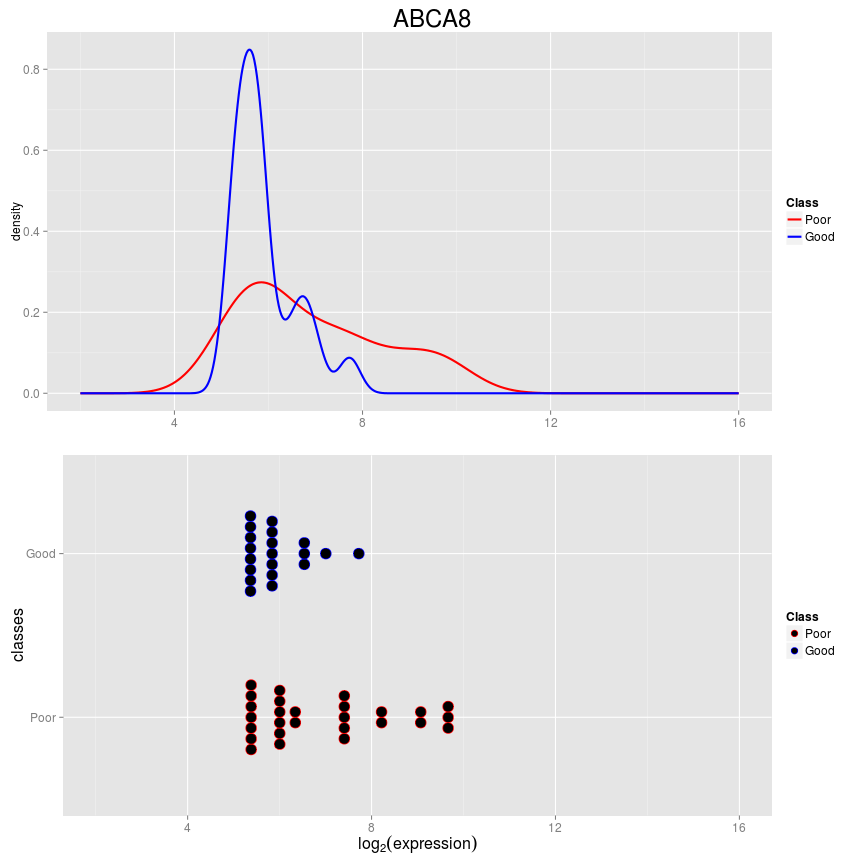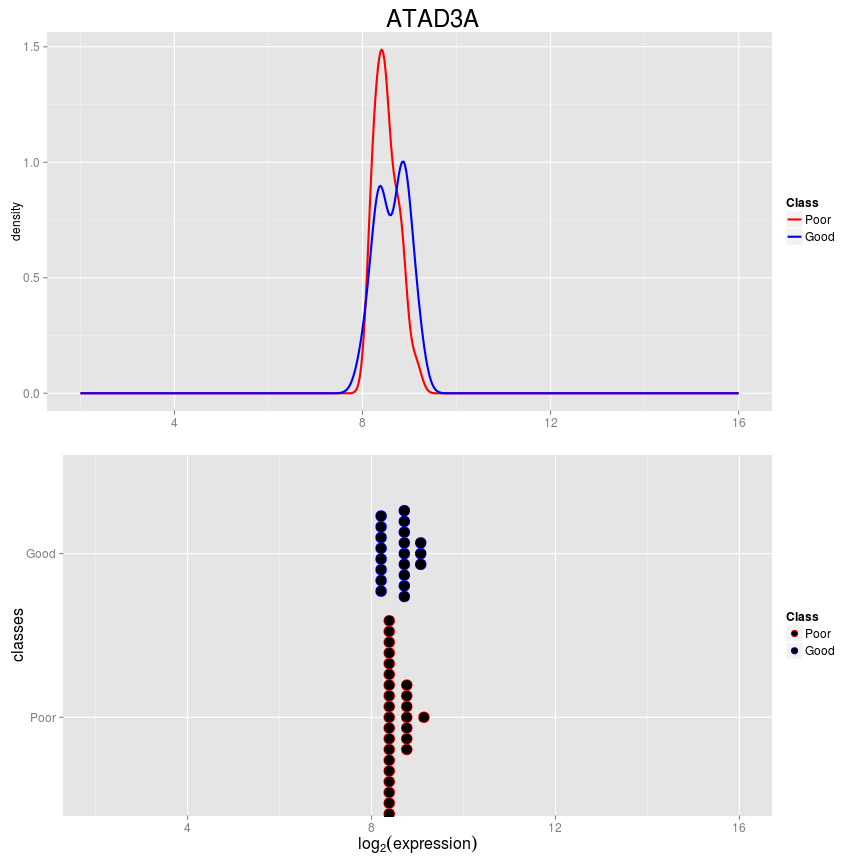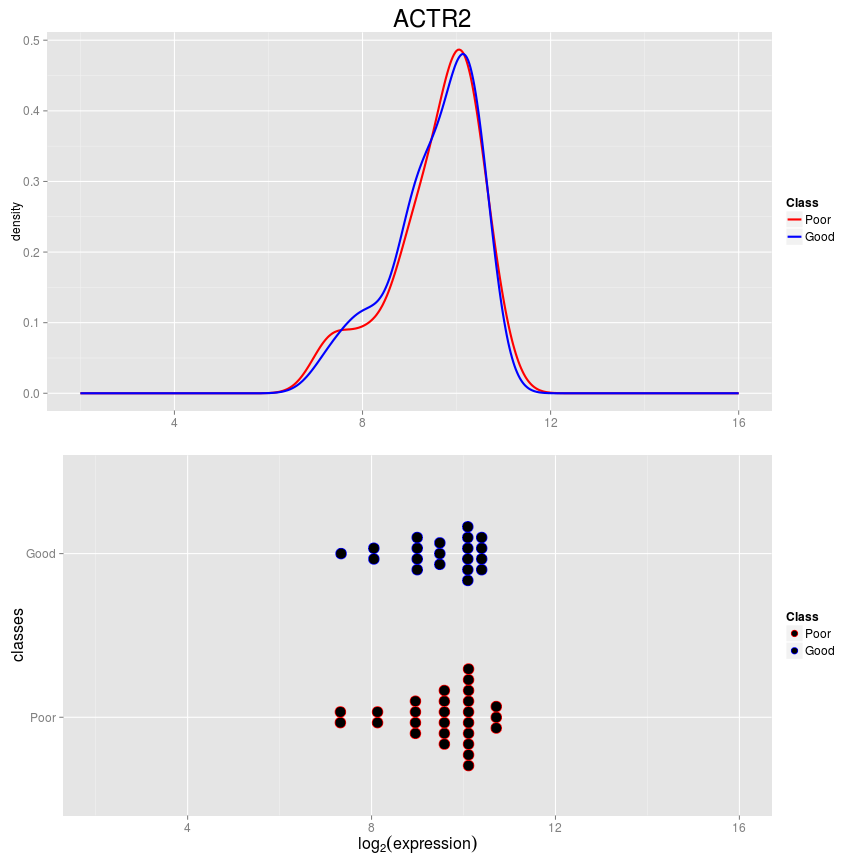Calculate the balanced error rate for differential deviation.

``````DVresults <- calcPerformance(DVresults, "balanced")
DVresults
``````
``````## An object of class 'ClassifyResult'.
## Dataset Name: Ovarian Cancer.
## Classification Name: Differential Deviation.
## Validation: 4 fold cross-validation of 2 resamples.
## Predictions: List of data frames of length 2.
## Features: List of length 2 of lists of length 4 of row indices.
## Performance Measures: Balanced Error Rate.
``````
``````performance(DVresults)
``````
``````## \$`Balanced Error Rate`
##  0.4332707 0.3790909
``````

The errors are reasonable.

ClassifyR has one feature selection function for differential deviation, leveneSelection, and one classifier, fisherDiscriminant. Fisher's LDA is suitable for the absolute value of expression values subtracted from a location, because it does not assume normality, unlike ordinary LDA.

### Differential Distribution

Differential distribution describes classification based on differences in either the location, scale, or both aspects of a distribution.

Kullback-Leibler divergence will be used for feature selection and a naive Bayes classifier will fit a density to expression values of a gene, for each class. The prediction is then the differences in density between classes, scaled for the number of samples that were in each class in the training set, summed for all selected probes.

``````DDresults <- runTests(ovarSet, "Ovarian Cancer", "Differential Distribution",
validation = "bootstrap", resamples = 2, folds = 2,
params = list(SelectionParams(KullbackLeiblerSelection, resubstituteParams = ResubstituteParams(nFeatures = c(25, 50, 75, seq(100, 1000, 100)), performanceType = "balanced", better = "lower")),
TrainParams(naiveBayesKernel, FALSE, doesTests = TRUE),
PredictParams(predictor = function(){}, FALSE, FALSE, getClasses = function(result) result, weighted = "weighted")),
verbose = 1)
DDresults
``````
``````## An object of class 'ClassifyResult'.
## Dataset Name: Ovarian Cancer.
## Classification Name: Differential Distribution.
## Validation: 2 fold cross-validation of 2 resamples.
## Predictions: List of data frames of length 2.
## Features: List of length 2 of lists of length 2 of row indices.
## Performance Measures: None calculated yet.
``````

Since naiveBayesKernel does both training and testing, an empty function is specified as the predictor function for the PredictParams constructor.

The top five probes selected for the first resampling and first fold are visualised.

``````plotFeatureClasses(ovarSet, features(DDresults)[][][1:5])
``````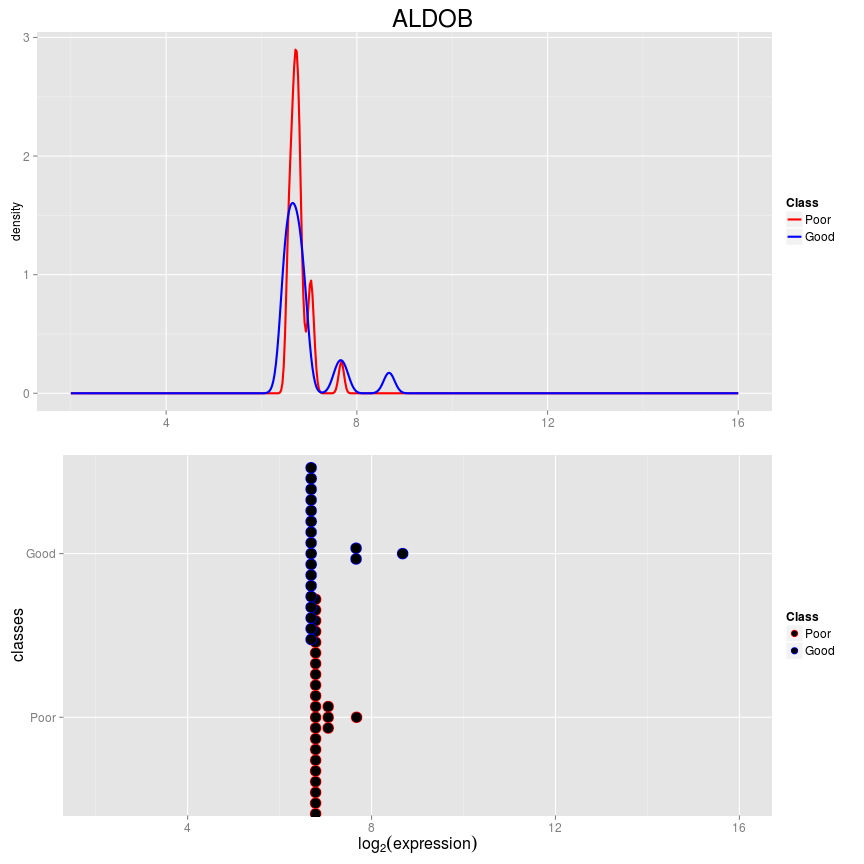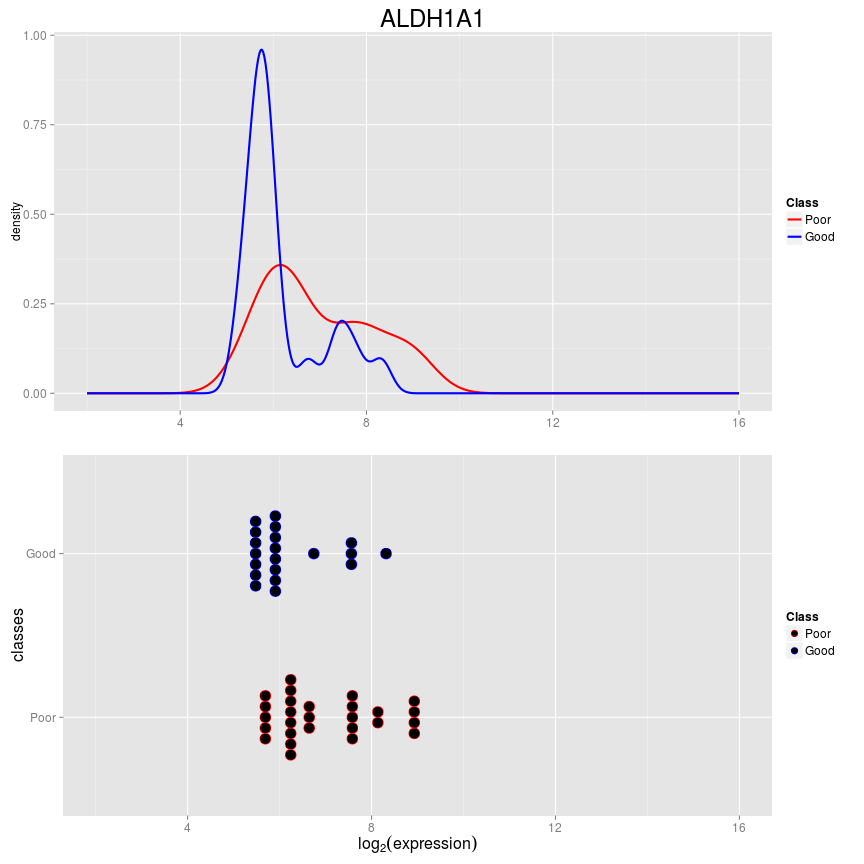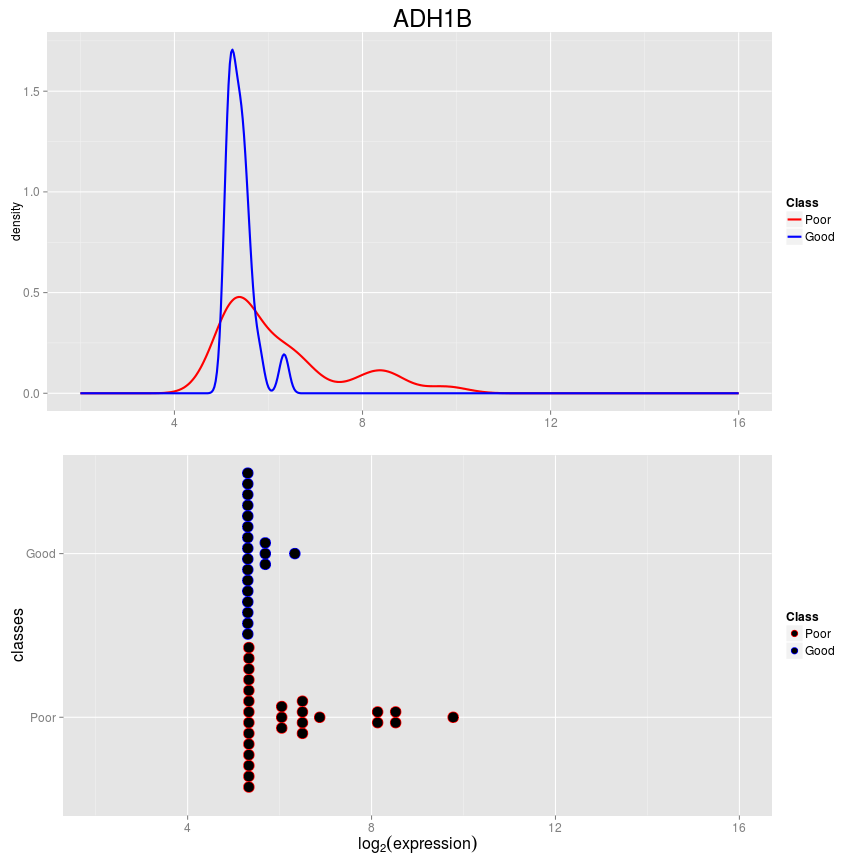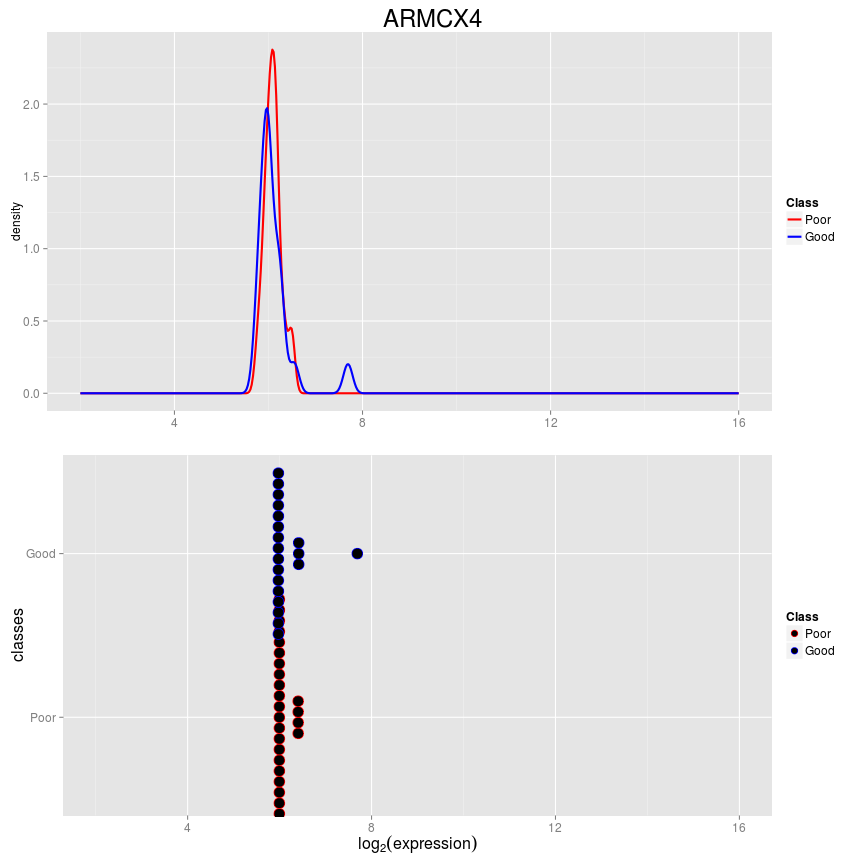Calculate the balanced error rate for differential distribution.

``````DDresults <- calcPerformance(DDresults, "balanced")
DDresults
``````
``````## An object of class 'ClassifyResult'.
## Dataset Name: Ovarian Cancer.
## Classification Name: Differential Distribution.
## Validation: 2 fold cross-validation of 2 resamples.
## Predictions: List of data frames of length 2.
## Features: List of length 2 of lists of length 2 of row indices.
## Performance Measures: Balanced Error Rate.
``````
``````performance(DDresults)
``````
``````## \$`Balanced Error Rate`
##  0.5000000 0.4754545
``````

The error rates are higher than for deviation or expression.

ClassifyR has four feature selection functions for differential distribution :

• Likelihood ratio statistic.
• Kolmogorov-Smirnov distance.
• Kullback-Leibler distance.
• Differences of Means/Medians and Deviations (DMD).

There are also two classifiers :

• Naive Bayes.
• Mixtures of normals.

### Comparison of Methods

The errorMap function allows the visual comparison of error rates from different ClassifyResult objects.

``````library(grid)
resultsList <- list(Expression = DEresults, Variability = DVresults)
errorPlot <- errorMap(resultsList)
``````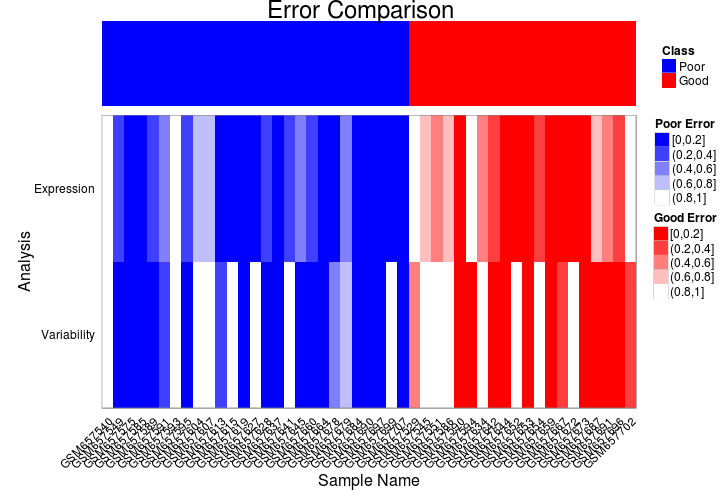### Conclusion

When many replicates per class are available, differential variability or distribution classification may have better prediction perfomance than traditional differential expression analysis. Judging by feature selection, the probes chosen for their differential distribution have much stronger differences than those for expression.

## Rules for New Functions

Transform Function : The first argument must be an ExpressionSet. Other arguments may be anything. The argument verbose is sent from runTest, so it must handle it. It returns an ExpressionSet of the same dimensions as the input ExpressionSet. Selection Function : The first argument must be an ExpressionSet. It must also handle trainParams, predictParams, and verbose, even if it does not use them. It returns a list of length 2. The first element has features ranked from best to worst. The second has information about the features that were selected to be used in classifier training. Training Function : The first argument must be a matrix. This is because most other R classifiers on CRAN take matrices. This avoids having to write interfaces for them. Other arguments may be anything. The argument verbose is sent from runTest, so it must handle it. It returns a classifier. Prediction Function : The first argument must be a trained model that was generated by the training step. The second argument must be a matrix of test data. Other arguments may be anything. The argument verbose is sent from runTest, so it must handle it. It returns an object containing predictions.

## References

Strbenac D., Ormerod, J. T., and Yang, J. ClassifyR: An R Package for Reproducible Classification with Applications to Differential Variability and Differential Distributions. In preparation.